# ML Aggarwal Solutions Class 10 Maths Chapter 13 Similarity

Exercise 13.1

1. State which pairs of triangles in the figure given below are similar. Write the similarity rule used and also write the pairs of similar triangles in symbolic form (all lengths of sides are in cm):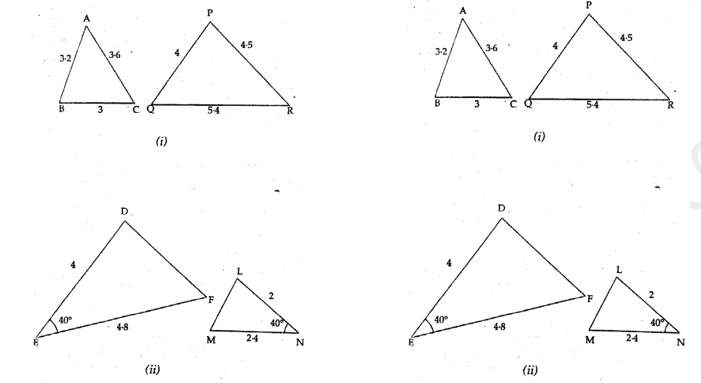Solution:-
(i) From the given figure
We have ΔABC and ΔPQR
AB/PQ = 3.2/4
= 32/40
Divide both numerator and denominator by 8 we get,
= 4/5
AC/PR = 3.6/4.5
= 36/45
Divide both numerator and denominator by 9 we get,
= 4/5
BC/QR = 3/5.4
= 30/54
Divide both numerator and denominator by 6 we get,
= 5/9
By comparing all the results, the side are not equal.
Therefore, the triangles are not equal.
(ii) From the given figure
We have ΔDEF and ΔLMN
∠E = ∠N = 40°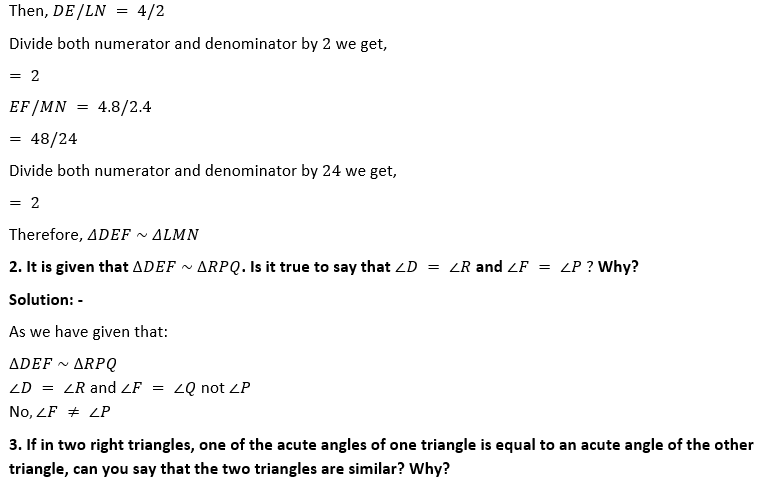Solution:-
From the figure, two line segments are intersecting each other at P.
In ΔBCP and ΔDPE
5/10 = 6/12
Dividing LHS and RHS by 2 we get,
½ = ½
Therefore, ΔBCD ~ ΔDEP

4. It is given that ∆ABC ~ ∆EDF such that AB = 5 cm,AC = 7 cm,DF = 15 cm and DE = 12 cm.
Find the lengths of the remaining sides of the triangles.
Solution:-
As we have given that: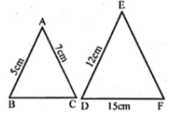As per the dimensions give in the questions,
ΔDEF ~ ΔLMN
So, AB/ED = AC/EF = BC/DF

Consider AB/ED = AC/EF
5/12 = 7/EF
By cross multiplication, we get
EF = (7 × 12)/5
EF = 16.8 cm
Now, consider AB/ED = BC/DF
5/12 = BC/15
BC = (5 × 15)/12
BC = 75/12
BC = 6.25
Hence, the length of BC=6.25 cm

5. (a) If ∆ABC ~ ∆DEF,AB = 4 cm,DE = 6 cm,EF = 9 cm and FD = 12 cm, then find the perimeter of ∆ABC.
Solution:-
As per the dimensions give in the questions,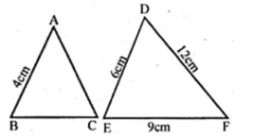Now, to find out the perimeter of ΔABC
Let ΔABC ~ ΔDEF
So, AB/DE = AC/DF = BC/EF
Consider, AB/DE = AC/DE
4/6 = AC/12
By cross multiplication we get,
AC = (4 × 12)/6
AC = 48/6
AC = 8 cm
Then, consider AB/DE = BC/EF
4/6 = BC/9
BC = (4 × 9)/6
BC = 36/6
BC = 6 cm
Therefore, the perimeter of ΔABC = AB + BC + AC
= 4 + 6 + 8
= 18 cm
Hence, the perimeter of ∆ ABC is 18cm

(b) If ∆ABC ~ ∆PQR, Perimeter of ∆ABC = 32 cm, perimeter of ∆PQR = 48 cm and PR = 6 cm, then find the length of AC.
Solution:-
We have given that:
∆ABC ~ ∆PQR
Perimeter of ∆ABC = 32 cm
Perimeter of ∆PQR = 48 cm
So, AB/PQ = AC/PR = BC/QR
Then, perimeter of ∆ABC/perimeter of ∆PQR = AC/PR
32/48 = AC/6
AC = (32 × 6)/48
AC = 4
Therefore, the length of AC = 4 cm.

6. Calculate the other sides of a triangle whose shortest side is 6 cm and which is similar to a triangle whose sides are 4 cm,7 cm and 8 cm.
Solution:-
Let us assume that, ∆ABC ~ ∆DEF∆ABC is BC = 6cm
∆ABC ~ ∆DEF
So, AB/DE = BC/EF = AC/DF
Consider AB/DE = BC/EF
AB/8 = 6/4
AB = (6 × 8)/4
AB = 48/4
AB = 12
Now, consider BC/EF = AC/DF
6/4 = AC/7
AC = (6 × 7)/4
AC = 42/4
AC = 21/2
AC = 10.5 cm
Hence, the other side of a triangle is 10.5 cm

7. (a) In the figure given below, AB || DE,AC = 3 cm,CE = 7.5 cm and BD = 14 cm. Calculate CB and DC.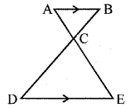Solution:-
From the question it is given that,
AB||DE
AC = 3 cm
CE = 7.5 cm
BD = 14 cm
From the figure,
∠ACB = ∠DCE [because vertically opposite angles]
∠BAC = ∠CED [alternate angles]
Then, ∆ABC ~ ∆CDE
So, AC/CE = BC/CD
3/7.5 = BC/CD
By cross multiplication we get,
7.5BC = 3CD
Let us assume BC = x and CD = 14 – x
7.5 × x = 3 × (14 – x)
7.5x = 42 – 3x
7.5x + 3x = 42
10.5x = 42
x = 42/10.5
x = 4
Therefore, BC = x = 4 cm
CD = 14 – x
= 14 – 4
= 10 cm
Hence, the length of BC=4cm and DC=10cm

(b) In the figure (2) given below, CA || BD, the lines AB and CD meet at G.
(i) Prove that ∆ACO ~ ∆BDO.
(ii) If BD = 2.4 cm,OD = 4 cm,OB = 3.2 cm and AC = 3.6 cm, calculate OA and OC.Solution:-
We have given that:
(i) We have to prove that, ∆ACO ~ ∆BDO.
So, from the figure
Consider ∆ACO and ∆BDO
Then,
∠AOC = ∠BOD [from vertically opposite angles]
∠A = ∠B
Therefore, ∆ACO = ∆BDO
Hence proved.
(ii) As we have given that,
BD = 2.4 cm,OD = 4 cm,OB = 3.2 cm,AC = 3.6 cm,
∆ACO ~ ∆BOD
So, AO/OB = CO/OD = AC/BD
Consider AC/BD = AO/OB
3.6/2.4 = AO/3.2
AO = (3.6 × 3.2)/2.4
AO = 4.8 cm
Now, consider AC/BD = CO/OD
3.6/2.4 = CO/4
CO = (3.6 × 4)/2.4
CO = 6 cm
Hence, length of AO=4.8 cm and CO=6cm

8. (a) In the figure
(i) given below, ∠P = ∠RTS.
Prove that ∆RPQ ~ ∆RTS.Solution:-
We have given that:
From the given figure, ∠P = ∠RTS
So we have to prove that ∆RPQ ~ ∆RTS
In ∆RPQ and ∆RTS
∠R = ∠R (common angle for both triangle)
∠P = ∠RTS (from the question)
∆RPQ ~ ∆RTS
BD = 4.5/2
BD = 2.25 cm

(b) In the figure(2) given below,
(i)  Prove that ∆s ABC and AED are similar.
(ii) If AE=3cm,BD=1cm and AB=6cm, calculate AC.Solution:-
As per given information,
(i) ∠A = ∠A (common angle for both triangles)
Therefore, ∆ABC ~ ∆AED
(ii) from (i) proved that, ∆ABC ~ ∆AED
So, BC/DE = AB/AE = AC/AD
= 6 – 1 = 5
6/3 = AC/5
AC = (6 × 5)/3
AC = 30/3
AC = 10 cm
Hence, the length of AC=10 cm

(c) In the figure (3) given below, ∠PQR = ∠PRS. Prove that triangles PQR and PRS are similar. If PR = 8 cm,PS = 4 cm, calculate PQ.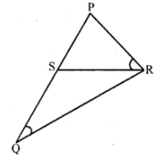Solution:-
We have given that:
From the figure,
∠P = ∠P (common angle for both triangles)
∠PQR = ∠PRS [from the question]
So, ∆PQR ~ ∆PRS
Then, PQ/PR = PR/PS = QR/SR
Consider PQ/PR = PR/PS
PQ/8 = 8/4
PQ = (8 × 8)/4
PQ = 64/4
PQ = 16 cm
Hence, the length of PQ=16cm

10. In the given figure, ABC is a triangle in which AB = AC.P is a point on the side BC such that PM ⊥ AB and PN ⊥ AC. Prove that BM x NP = CN× MP.Solution:-
As we have given that,
ABC is a triangle in which AB = AC.
P is a point on the side BC such that PM ⊥ AB and PN ⊥ AC.
We have to prove that, BM x NP = CN x MP
Consider the ∆ABC
AB = AC … [from the question]
∠B = ∠C … [angles opposite to equal sides]
Then, consider ∆BMP and ∆CNP
∠M = ∠N
Therefore, ∆BMP ~ ∆CNP
So, BM/CN = MP/NP
By cross multiplication we get,
BM × NP = CN × MP
Hence it is proved.

11. Prove that the ratio of the perimeters of two similar triangles is the same as the ratio of their corresponding sides.
Solution:-
We have given that:
Consider the two triangles, ∆MNO and ∆XYZ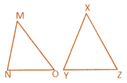From the question it is given that, two triangles are similar triangles
So, ∆MNO ~ ∆XYZ
If two triangles are similar, the corresponding angles are equal and their corresponding sides are proportional.
MN/XY = NO/YZ = MO/XZ
Perimeter of ∆MNO = MN + NO + MO
Perimeter of ∆XYZ = XY + YZ + XZ
Therefore, (MN/XY = NO/YZ = MO/XZ) = (MN/XY + NO/YZ + MO/XZ)
= Perimeter of ∆MNO/perimeter of ∆XYZ

12. In the adjoining figure, ABCD is a trapezium in which AB || DC. The diagonals AC and BD intersect at O. Prove that AO/OC = BO/OD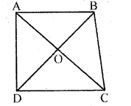Using the above result, find the values of x if OA = 3x – 19,OB = x – 4,OC = x – 3 and OD = 4.
Solution:-
We have given that:
From the given figure, ABCD is a trapezium in which AB || DC,
The diagonals AC and BD intersect at O.
So we have to prove that, AO/OC = BO/OD
Consider the ∆AOB and ∆COD,
∠AOB = ∠COD … [vertically opposite angles]
∠OAB = ∠OCD
Therefore, ∆AOB ~ ∆COD
So, OA/OC = OB/OD
Now by using above result we have to find the value of x if OA = 3x – 19,OB = x – 4,OC = x – 3 and OD = 4.
OA/OC = OB/OD
(3x – 19)/(x – 3) = (x – 4)/4
By cross multiplication we get,
(x – 3) (x – 4) = 4(3x – 19)
X – 4x – 3x + 12 = 12x – 76
X2– 7x + 12 – 12x + 76 = 0
X2– 19x + 88 = 0
X2– 8x – 11x + 88 = 0
X(x – 8) – 11(x – 8) = 0
(x – 8) (x – 11) = 0
Take x – 8 = 0
X = 8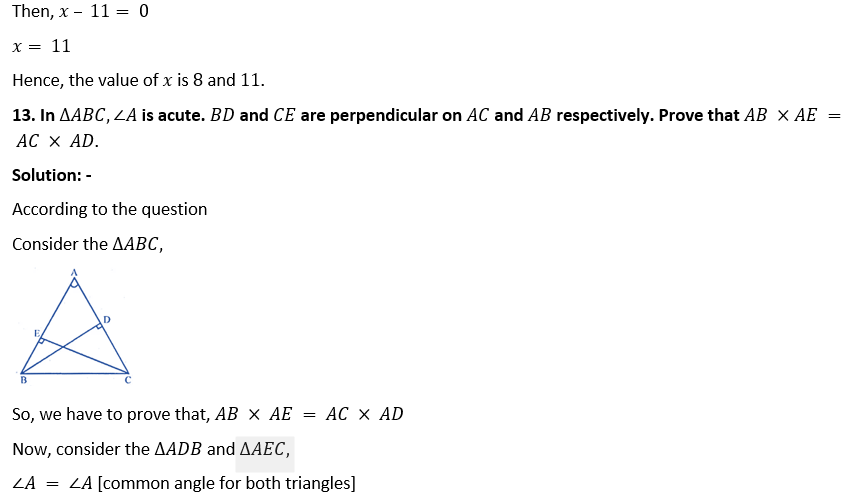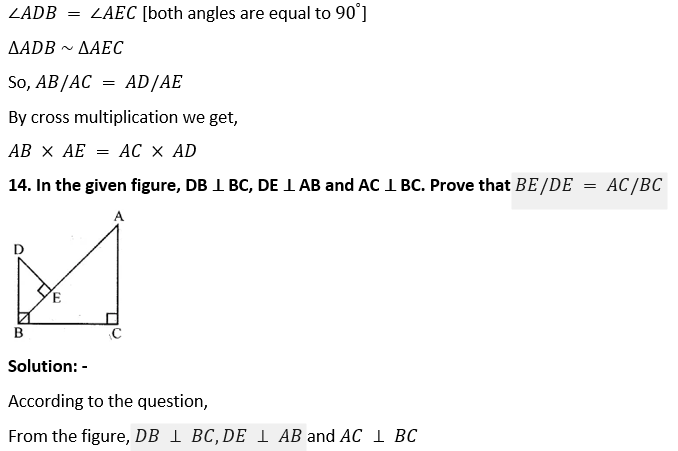We have to prove that, BE/DE = AC/BC
Consider the ∆ABC and ∆DEB,
∠C = 90°
∠A + ∠ABC = 90°[from the figure equation (i)]
Now in ∆DEB
∠DBE + ∠ABC = 90°[from the figure equation (ii)]
From equation (i), we get
∠A = ∠DBE
Then, in ∆ABC and ∆DBE
∠C = ∠E [both angles are equal to 90°
So, ∆ABC ~ ∆DBE
Therefore, AC/BE = BC/DE
By cross multiplication, we get
AC/BC = BE/DE
Hence proved

15. (a) In the figure (1) given below, E is a point on the side AD produced of a parallelogram ABCD and BE intersects CD at F. show that ∆ABE ~ ∆CFB.
Solution:-
According to the question,
From the figure, ABCD is a parallelogram,
Then, E is a point on AD and produced and BE intersects CD at F.
We have to prove that ∆ABE ~ ∆CFB
Consider ∆ABE and ∆CFB
∠A = ∠C [opposite angles of a parallelogram]
∠ABE = ∠BFC [alternate angles are equal]
∆ABE ~ ∆CFB
Hence proved

(b) In the figure (2) given below, PQRS is a parallelogram; PQ = 16 cm,QR = 10 cm.L is a point on PR such that RL∶ LP = 2∶ 3.QL produced meets RS at M and PS produced at N.
(i) Prove that triangle RLQ is similar to triangle PLN. Hence, find PN.
Solution:-
According to the question,
Consider the ∆RLQ and ∆PLN,
∠RLQ = ∠NLP [vertically opposite angles are equal]
∠RQL = ∠LNP [alternate angle are equal]
Therefore, ∆RLQ ~ ∆PLN
So, QR/PN = RL/LP = 2/3
QR/PN = 2/3
10/PN = 2/3
PN = (10 × 3)/2
PN = 30/2
PN = 15 cm
Hence, the length of PN=15cm

(ii) Name a triangle similar to triangle RLM. Evaluate RM.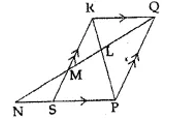Solution:-
According to the given figure,
Consider ∆RLM and ∆QLP
Then, ∠RLM = ∠QLP [vertically opposite angles are equal]
∠LRM = ∠LPQ [alternate angles are equal]
Therefore, ∆RLM ~ ∆QLP
Then, RM/PQ = RL/LP = 2/3
So, RM/16 = 2/3
RM = (16 × 2)/3
RM = 32/3
RM = 10 2/3

16. The altitude BN and CM of ∆ABC meet at H. Prove that
(i) CN × HM = BM × HN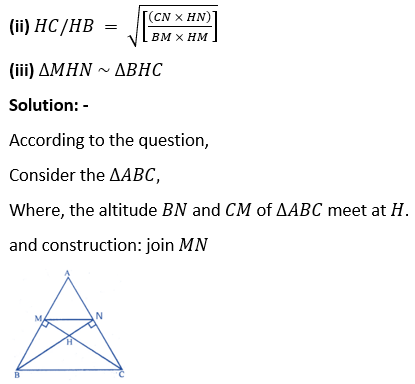(i) We have to prove that, CN × HM = BM × HN
In ∆BHM and ∆CHN
∠BHM = ∠CHN [because vertically opposite angles are equal]
∠M = ∠N [both angles are equal to 90o]
Therefore, ∆BHM ~ ∆CHN
So, HM/HN = BM/CN = HB/HC
Then, by cross multiplication we get
CN × HM = BM × HN(iii) Now consider ∆MHN and ∆BHC
∠MHN = ∠BHC [because vertically opposite angles are equal]
∠MNH = ∠HBC [because alternate angles are equal]
Therefore, ∆MHN ~ ∆BHC

17. In the given figure, CM and RN are respectively the medians of ∆ABC and ∆PQR. If ∆ABC ~ ∆PQR, prove that:
(i) ∆AMC ~ ∆PQR
(ii) CM/RN = AB/PQ
(iii) ∆CMB ~ ∆RNQSolution:-
As per the given information:
CM and RN are respectively the medians of ∆ABC and ∆PQR.
(i) We have to prove that, ∆AMC ~ ∆PQR
Consider the ∆ABC and ∆PQR
As ∆ABC ~ ∆PQR
∠A = ∠P,∠B = ∠Q and ∠C = ∠R
And also corresponding sides are proportional
AB/PQ = BC/QR = CA/RP
Then, consider the ∆AMC and ∆PNR,
∠A = ∠P
AC/PR = AM/PN
Because, AB/PQ = ½ AB/½PQ
AB/PQ = AM/PN
Therefore, ∆AMC ~ ∆PNR
(ii) From solution(i) CM/RN = AM/PN
CM/RN = 2AM/2PN
CM/RN = AB/PQ
(iii)Now consider the ∆CMB and ∆RNQ
∠B = ∠Q
BC/QP = BM/QN
Therefore, ∆CMB ~ ∆RNQ

18. In the adjoining figure, medians AD and BE of ∆ABC meet at the point G, and DF is drawn parallel to BE. Prove that
(i) EF = FC
(ii) AG∶ GD = 2∶ 1Solution:-
According to the question,
Medians AD and BE of ∆ABC meet at the point G, and DF is drawn parallel to BE.
(i) We have to prove that, EF = FC
From the figure, D is the midpoint of BC and also DF parallel to BE.
So, F is the midpoint of EC
Therefore, EF = FC
= ½ EC
EF = ½ AE
Hence proved
(ii) Now consider the ∆AGE and ∆ADF
Then, (BG or GE) ||DF
So, AG/GD = AE/EF
AG/GD = 1/½
AG/GD = 1 × (2/1)
Therefore, AG: GD = 2: 1
Hence proved

19.(a) In the figure given below, AB,EF and CD are parallel lines. Given that AB =15 cm,EG = 5 cm,GC = 10 cm and DC = 18 cm. Calculate
(i) EF
(ii) AC.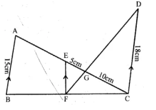Solution:-
As per the given information,
AB,EF and CD are parallel lines.
(i) Consider the ∆EFG and ∆CGD
∠EGF = ∠CGD [Because vertically opposite angles are equal]
∠FEG = ∠GCD [alternate angles are equal]
Therefore, ∆EFG ~ ∆CGD
Then, EG/GC = EF/CD
5/10 = EF/18
EF = (5 × 18)/10
Hence, the length of EF = 9 cm
(ii) Now, consider the ∆ABC and ∆EFC
EF ||AB
So, ∆ABC ~ ∆EFC
Then, AC/EC = AB/EF
AC/(5 + 10) = 15/9
AC/15 = 15/9
AC = (15 × 15)/9
Therefore, AC = 25 cm
Hence, the length of AC = 25 cm.

(b) In the figure given below, AF,BE and CD are parallel lines. Given that AF = 7.5 cm,CD = 4.5 cm,ED = 3 cm,BE = x and AE = y. Find the values of x and y.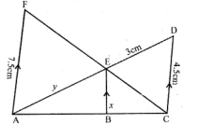Solution:-
We have given that:
From the figure, AF,BE and CD are parallel lines.
Consider the ∆AEF and ∆CED
∠AEF and ∠CED [because vertically opposite angles are equal]
∠F = ∠C [alternate angles are equal]
Therefore, ∆AEF ~ ∆CED
So, AF/CD = AE/ED
7.5/4.5 = y/3
By cross multiplication,
y = (7.5 × 3)/4.5
y = 5 cm
Hence, the value of y=5cm
So, similarly in ∆ACD,BE ||CD
Therefore, ∆ABE ~ ∆ACD
x/CD = y/y + 3
x/4.5 = 5/(5 + 3)
x/4.5 = 5/8
x = (4.5 × 5)/8
x = 22.5/8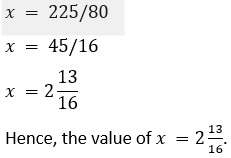21. In the given figure, ∠A = 90° and AD ⊥ BC If BD = 2 cm and CD = 8 cm, find AD.Solution:-
From the given figure, consider ∆ABC,
So, ∠A = 90°
∠BAC = 90°
Then, ∠BAD + ∠DAC = 90° … [equation (i)]
So, ∠DCA + ∠DAC = 90°… [equation (ii)]
From equation (i) and equation (ii)
We have,
∠BAD + ∠DAC = ∠DCA + ∠DAC
∠BAD = ∠DCA … [equation (iii)]
∠BDA = ∠ADC … [both the angles are equal to 90°
∠BAD = ∠DCA … [from equation (iii)]
Because, corresponding sides of similar triangles are proportional
By cross multiplication we get,
AD^2  = 2 × 8 = 16

22. A 15 metres high tower casts a shadow of 24 metres long at a certain time and at the same time, a telephone pole casts a shadow 16 metres long. Find the height of the telephone pole.
Solution:-
As we have given that,
Height of a tower PQ = 15m
It’s shadow QR = 24 m
Let us assume the height of a telephone pole MN = x
It’s shadow NO = 16 m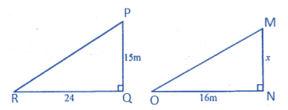At the same time, we have given that
∆PQR ~ ∆MNO
Therefore, PQ/MN = ON/RQ
15/x = 24/16
By cross multiplication we get,
x = (15 × 16)/24
x = 240/24
x = 10
Hence, the height of pole = 10 m.

23. A street light bulb is fixed on a pole 6 m above the level of street. If a woman of height casts a shadow of 3 m, find how far she is away from the base of the pole?
Solution:-
As per given information
Height of pole (PQ) = 6m
Height of a woman (MN) = 1.5m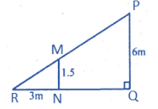Therefore, pole and woman are standing in the same line
PM ||MR
∆PRQ ~ ∆MNR
So, RQ/RN = PQ/MN
(3 + x)/3 = 6/1.5
(3 + x)/3 = 60/15
(3 + x)/3 = 4/1
(3 + x) = 12
x = 12 – 3
x = 9m
Hence, she is 9m away from the pole.

Exercise 13.2

1. (a) In the figure (i) given below if DE || BG,AD = 3 cm,BD = 4 cm and BC = 5 cm. Find (i) AE∶ EC (ii) DE.Solution:-
According to the question,
DE || BG,AD = 3 cm,BD = 4 cm and BC = 5 cm
(i) AE: EC
AE/EC = ¾
AE: EC = 3: 4
Hence, AE:EC=3:4
(ii) According to the question,
∠D = ∠B
∠E = ∠C
DE/5 = 3/(3 + 4)
DE/5 = 3/7
DE = (3 × 5)/7
DE = 15/7
DE =2 1/7
Hence, the length of DE=2 1/7

(b) In the figure (ii) given below, PQ || AC,AP = 4 cm,PB = 6 cm and BC = 8 cm. Find CQ and BQ.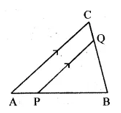Solution:-
From the figure,
PQ || AC,AP = 4 cm,PB = 6 cm and BC = 8 cm
∠BQP = ∠BCA … [because alternate angles are equal]
Also, ∠B = ∠B … [common for both the triangles]
Therefore, ∆ABC ~ ∆BPQ
Then, BQ/BC = BP/AB = PQ/AC
BQ/BC = 6/(6 + 4) = PQ/AC
BQ/BC = 6/10 = PQ/AC
BQ/8 = 6/10 = PQ/AC … [because BC = 8 cm given]
Now, BQ/8 = 6/10
BQ = (6/10) ×8
BQ = 48/10
BQ = 4.8 cm
Hence, the length of BQ=4.8cm
Also, CQ = BC – BQ
CQ = (8 – 4.8) cm
CQ = 3.2cm
Hence, the length of CQ=3.2cm

(c) In the figure (iii) given below, if XY || QR,PX = 1 cm,QX = 3 cm,YR = 4.5 cm and QR = 9 cm, find PY and XY.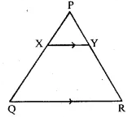Solution:-
According to the question,
From the given figure,
XY || QR,PX = 1 cm,QX = 3 cm,YR = 4.5 cm and QR = 9 cm,
So, PX/QX = PY/YR
1/3 = PY/4.5
By cross multiplication we get,
(4.5 × 1)/3 = PY
PY = 45/30
PY = 1.5
Hence, the value of PY=1.5
Then, ∠X = ∠Q
∠Y = ∠R
So, ∆PXY ~ ∆PQR
Therefore, XY/QR = PX/PQ
XY/9 = 1/(1 + 3)
XY/9 = ¼
XY = 9/4
XY = 2.25
Hence, the value of XY=2.25

2. In the given figure, DE || BC.
(i) If AD = x,DB = x – 2,AE = x + 2 and EC = x – 1, find the value of x.
(ii) If DB = x – 3,AB = 2x,EC = x – 2 and AC = 2x + 3, find the value of x.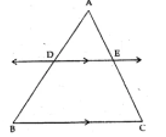Solution:-
(i) According to the question
From the figure, we have given that,
Consider the ∆ABC,
x/(x – 2) = (x + 2)/(x – 1)
By cross multiplication we get,
X(x – 1) = (x – 2) (x + 2)
x2  – x = x2– 4
-x = -4
x = 4
Hence, value of x=4.
(ii) According to the question,
DB = x – 3,AB = 2x,EC = x – 2 and AC = 2x + 3
Consider the ∆ABC,
2x/(x – 2) = (2x + 3)/(x – 3)
By cross multiplication we get,
2x(x – 2) = (2x + 3) (x – 3)
2x2  – 4x = 2x2  – 6x + 3x – 9
2x2  – 4x – 2x2  + 6x – 3x = -9
-7x + 6x = -9
-x = – 9
x = 9
Hence, the value of x=9.

3. E and F are points on the sides PQ and PR respectively of a ∆PQR. For each of the following cases, state whether EF || QR:
(i) PE = 3.9 cm,EQ = 3 cm,PF = 8 cm and RF = 9 cm.
Solution:-
As we have given that:
From the given dimensions,
Consider the ∆PQRSo, PE/EQ = 3.9/3
= 39/30
= 13/10
Then, PF/FR = 8/9
By comparing both the results,
13/10 ≠ 8/9
Therefore, PE/EQ ≠ PF/FR
Hence, EF is not parallel to QR

(ii) PQ = 1.28 cm,PR = 2.56 cm,PE = 0.18 cm and PF = 0.36 cm.
Solution:-
We have given that:
From the dimensions given in the question,
Consider the ∆PQRSo, PQ/PE = 1.28/0.18
= 128/18
= 64/9
Then, PR/PF = 2.56/0.36
= 256/36
= 64/9
By comparing both the results,
64/9 = 64/9
Therefore, PQ/PE = PR/PF
So, EF is parallel to QR.

4. A and B are respectively the points on the sides PQ and PR of a triangle PQR such that PQ = 12.5 cm,PA = 5 cm,BR = 6 cm and PB = 4 cm. Is AB || QR? Give reasons for your answer.
Solution:-
As per given information,
Consider the ∆PQRSo, PQ/PA = 12.5/5
= 2.5/1
PR/PB = (PB + BR)/PB
= (4 + 6)/4
= 10/4
= 2.5
By comparing both the results,
2.5 = 2.5
Therefore, PQ/PA = PR/PB
So, AB is parallel to QR.

5.(a) In figure (i) given below, DE || BC and BD = CE. Prove that ABC is an isosceles triangle.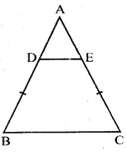Solution:-
We have given that:
DE || BC and BD = CE
So, we have to prove that ABC is an isosceles triangle.
Consider the triangle ABC,
Given, DB = EC … [equation (i)]
Then, AD = AE … [equation (ii)]
By adding equation (i) and equation (ii) we get,
AD + DB = AE + EC
So, AB = AC
Hence, ∆ABC is an isosceles triangle.

(b) In figure (ii) given below, AB || DE and BD || EF. Prove that DC² = CF × AC.Solution:-
From the figure,
We have given that, AB || DE and BD || EF.
To prove that, DC² = CF × AC
Consider the ∆ABC,
DC/CA = CE/CB … [equation (i)]
Now, consider ∆CDE
CF/CD = CE/CB … [equation (ii)]
From equation (i) and equation (ii),
DC/CA = CF/CD
DC/AC = CF/DC
By cross multiplication we get,
DC2  = CF× AC
Hence proved

6.(a) In the figure (i) given below, CD || LA and DE || AC. Find the length of CL if BE = 4 cm and EC = 2 cm.Solution:-
According to the question
We have given that, CD || LA and DE || AC,
Consider the ∆BCA,
BE/BC = BD/BA
By using the corollary of basic proportionality theorem,
BE/(BE + EC) = BD/AB
4/(4 + 2) = BD/AB … [equation (i)]
Then, consider the ∆BLA
BC/BL = BD/AB
By using the corollary of basic proportionality theorem,
6/(6 + CL) = BD/AB … [equation (ii)]
Now, combining the equation (i) and equation (ii), we get
6/(6 + CL) = 4/6
By cross multiplication we get,
6 x 6 = 4 x (6 + CL)
24 + 4CL = 36
4CL = 36 – 24
CL = 12/4
CL = 3 cm
Hence, the length of CL is 3 cm.

(b) In the give figure, ∠D = ∠E and AD/BD = AE/EC. Prove that BAC is an isosceles triangle.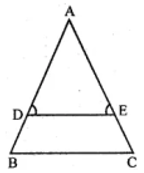Solution:-
From the given figure, ∠D = ∠E and AD/BD = AE/EC,
We have to prove that, BAC is an isosceles triangle
∠D = ∠E … [from the question]
AD = AE … [sides opposite to equal angles]
Consider the ∆ABC,
Then, AD/DB = AE/EC … [equation (i)]
Therefore, DE parallel to BC
DB = EC … [equation (ii)]
By adding equation (i) and equation (ii) we get,
AD + DB = AE + EC
AB = AC
Hence, ∆ABC is an isosceles triangle.
Hence proved.

7. In the adjoining given below, A,B and C are points on OP,OQ and OR respectively such that AB || PQ and AC || PR. show that BC || QR.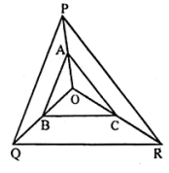Solution:-
As per given information,

Consider the ∆POQ
AB || PQ … [given]
So, OA/AP = OB/BQ … [equation (i)]
Then, consider the ∆OPR
AC || PR
OA/AP = OC/CR … [equation (ii)]
Now by comparing both equation (i) and equation (ii),
OB/BQ = OC/CR
Then, in ∆OQR
OB/BQ = OC/CR
Therefore, BC || QR
Hence proved.

8. ABCD is a trapezium in which AB || DC and its diagonals intersect each other at O. Using Basic Proportionality theorem, prove that AO/BO = CO/DO
Solution:-
As per given information,
We have given that
ABCD is a trapezium in which AB || DC and its diagonals intersect each other at O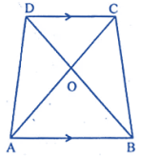Now consider the ∆OAB and ∆OCD,
∠AOB = ∠COD [because vertically opposite angles are equal]
∠OBA = ∠ODC [because alternate angles are equal]
∠OAB = ∠OCD [because alternate angles are equal]
Therefore, ∆OAB ~ ∆OCD
Then, OA/OC = OB/OD
AO/OB = CO/DO … [by alternate angles]
Hence proved.

9.(a) In the figure (1) given below, AB || CR and LM || QR.
(i) Prove that BM/MC = AL/LQ
(ii) Calculate LM∶ QR, given that BM∶ MC = 1∶ 2.Solution:-
We have given that: AB || CR and LM || QR
(i) We have to prove that, BM/MC = AL/LQ
Consider the ∆ARQ
LM || QR … [from the question]
So, AM/MR = AL/LQ … [equation (i)]
Now, consider the ∆AMB and ∆MCR
∠AMB = ∠CMR … [because vertically opposite angles are equal]
∠MBA = ∠MCR … [because alternate angles are equal]
Therefore, AM/MR = BM/MC … [equation (ii)]
From equation (i) and equation (ii) we get,
BM/MR = AL/LQ
Hence proved
(ii) Given, BM∶ MC = 1∶ 2
AM/MR = BM/MC
AM/MR = ½ … [equation (iii)]
LM || QR … [given from equation]
AM/MR = LM/QR … [equation (iv)]
AR/AM = QR/LM
(AM + MR)/AM = QR/LM
1 + MR/AM = QR/LM
1 + (2/1) = QR/LM
3/1 = QR/LM
LM/QR = 1/3
Hence, the ratio of LM: QR is 1: 3.

(b) In the figure (2) given below AD is bisector of ∠BAC. If AB = 6 cm,AC = 4 cm and BD = 3cm, find BC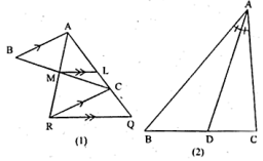Solution:-
As per given figure,
AB = 6 cm,AC = 4 cm and BD = 3cm
Construction, from C draw a straight line CE parallel to DA and join AE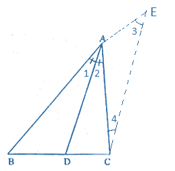∠1 = ∠2 … [equation (i)]
By construction CE || DE
So, ∠2 = ∠4 … [because alternate angles are equal] [equation (ii)]
Again by construction CE || DE
∠1 = ∠3 … [because corresponding angles are equal] [equation (iii)]
By comparing equation (i), equation (ii) and equation(iii) we get,
∠3 = ∠4
So, AC = AE … [equation (iv)]
Now, consider the ∆BCE,
CE || DE
BD/DC = AB/AE
BD/DC = AB/AC
3/DC = 6/4
By cross multiplication we get,
3 × 4 = 6 × DC
DC = (3 × 4)/6
DC = 12/6
DC = 2
Therefore, BC = BD + DC
= 3 + 2
= 5 cm
Hence, the value of BC=5cm

Exercise 13.3

1. Given that ∆s ABC and PQR are similar.
Find:
(i) The ratio of the area of ∆ABC to the area of ∆PQR if their corresponding sides are in the ratio 1∶ 3.
(ii) the ratio of their corresponding sides if area of ∆ABC : area of ∆PQR = 25∶ 36.
Solution:-
We have given that:
(i) The area of ∆ABC to the area of ∆PQR if their corresponding sides are in the ratio 1∶ 3
Then, ∆ABC ~ ∆PQR
area of ∆ABC/area of ∆PQR = BC2/QR2
So, BC∶ QR = 1∶ 3
Therefore, ∆ABC/area of ∆PQR = 12/32
= 1/9
Hence the ratio of the area of ∆ABC to the area of ∆PQR is 1: 9
(ii) The area of ∆ABC to the area of ∆PQR if their corresponding sides are in the ratio 25∶ 36
Then, ∆ABC ~ ∆PQR
area of ∆ABC/area of ∆PQR = BC2/QR2
area of ∆ABC/area of ∆PQR = BC2/QR2  = 25/36
= (BC/QR)2  = (5/6)2
BC/QR = 5/6
Hence the ratio of their corresponding sides is 5∶ 6

2. ∆ABC ~ DEF. If area of ∆ABC = 9 sq.cm., area of ∆DEF =16 sq.cm and BC = 2.1 cm., find the length of EF.
Solution:-
We have given that:
∆ABC ~ DEF
Area of ∆ABC = 9 sq.cm
Area of ∆DEF =16 sq.cm
We know that,
area of ∆ABC/area of ∆DEF = BC2/EF2
area of ∆ABC/area of ∆DEF = BC2/EF2
9/16 = BC2/EF2
9/16 = (2.1)2/x2
2.1/x = √9/√16
2.1/x = ¾
By cross multiplication we get,
2.1 × 4 = 3 × x
8.4 = 3x
x = 8.4/3
x = 2.8
Hence, the length of EF = 2.8 cm

3. ∆ABC ~ ∆DEF. If BC = 3 cm,EF = 4 cm and area of ∆ABC = 54 sq.cm. Determine the area of ∆DEF.
Solution:-
As per given information,
∆ABC ~ ∆DEF
BC = 3 cm,EF = 4 cm
Area of ∆ABC = 54 sq.cm.
We know that,
Area of ∆ABC/ area of ∆DEF = BC2/EF2
54/area of ∆DEF = 32/42
54/area of ∆DEF = 9/16
By cross multiplication we get,
Area of ∆DEF = (54 × 16)/9
= 6 × 16
= 96 cm
Hence, the area of ∆DEF=96 cm

4. The area of two similar triangles are 36 cm² and 25 cm². If an altitude of the first triangle is 2.4 cm, find the corresponding altitude of the other triangle.
Solution:-
We have given that:
The area of two similar triangles are 36 cm² and 25 cm².
Let us assume ∆PQR ~ ∆XYZ,PM and XN are their altitudes.
So, area of ∆PQR = 36 cm2
Area of ∆XYZ = 25 cm2
PM = 2.4 cm
Assume XN = a
We know that,
area of ∆PQR/area of ∆XYZ = PM2/XN2
36/25 = (2.4)2/a2
By cross multiplication we get,
36a2  = 25 (2.4)2
a2 = 5.76 × 25/36
a2 = 144/36
a2 = 4
a = √4
a = 2 cm
Hence, the corresponding altitude of the other triangle XN = 2 cm.

5.(a) In the figure, (i) given below, PB and QA are perpendiculars to the line segment AB. If PO = 6 cm,QO = 9 cm and the area of ∆POB = 120 cm², find the area of ∆QOA.Solution:-
As per given information
PO = 6 cm,QO = 9 cm and the area of ∆POB = 120 cm²
From the figure,
Consider the ∆AOQ and ∆BOP,
∠OAQ = ∠OBP … [both angles are equal to 90o]
∠AOQ = ∠BOP … [because vertically opposite angles are equal]
Therefore, ∆AOQ ~ ∆BOP
Then, area of ∆AOQ/area of ∆BOP = OQ2/PO2
Area of ∆AOQ/120 = 92/62
Area of ∆AOQ/120 = 81/36
Area of ∆AOQ = (81 × 120)/36
Area of ∆AOQ = 270 cm2

b) In the figure (ii) given below, AB || DC.AO = 10 cm,OC = 5cm,AB = 6.5 cm and OD = 2.8 cm.
(i) Prove that ∆OAB ~ ∆OCD.
(ii) Find CD and OB.
(iii) Find the ratio of areas of ∆OAB and ∆OCD.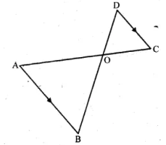Solution:-
As per given information,
AB || DC.AO = 10 cm,OC = 5cm,AB = 6.5 cm and OD = 2.8 cm
(i) We have to prove that, ∆OAB ~ ∆OCD
So, consider the ∆OAB and ∆OCD
∠AOB = ∠COD … [because vertically opposite angles are equal]
∠OBA = ∠OCD … [because alternate angles are equal]
Therefore, ∆OAB ~ ∆OCD … [from AAA axiom]
(ii) Consider the ∆OAB and ∆OCD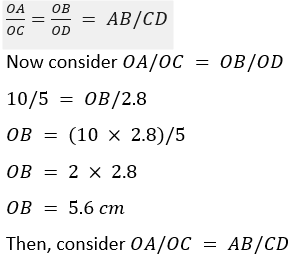10/5 = 6.5/CD
CD = (6.5 × 5)/10
CD = 32.5/10
CD = 3.25 cm
Hence, the length of CD=3.25cm
(iii) We have to find the ratio of areas of ∆OAB and ∆OCD.
From (i) we proved that, ∆OAB ~ ∆OCD
Then, area of (∆OAB)/area of ∆OCD
AB2/CD2  = (6.5)2/(3.25)2
= (6.5 × 6.5)/(3.25 × 3.25)
= 2 × 2/1
= 4/1
Therefore, the ratio of areas of ∆OAB and ∆OCD = 4: 1.

6.(a) In the figure (i) given below, DE || BC.If DE = 6 cm,BC = 9 cm and area of ∆ADE = 28 sq.cm, find the area of ∆ABC.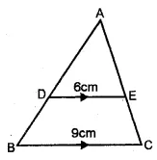Solution:-
As per given information,
DE || BC,DE = 6 cm,BC = 9 cm and area of ∆ADE = 28 sq.cm
From the fig, ∠D = ∠B and ∠E = ∠C … [corresponding angles are equal]
Now consider the ∆ADE and ∆ABC,
∠A = ∠A … [common angles for both triangles]
Then, area of ∆ADE/area of ∆ABC = (DE)2 /(BC)2
28/area of ∆ABC = (6)2/(9)2
28/area of ∆ABC = 36/81
area of ∆ABC = (28 × 81)/36
area of ∆ABC = 2268/36
area of ∆ABC = 63 cm2
Hence, area of ∆ABC=63cm2

(b) In the figure (ii) given below, DE || BC and AD∶ DB = 1∶ 2, find the ratio of the areas of ∆ADE and trapezium DBCE.Solution:-
We have given that: DE || BC and AD∶ DB = 1∶ 2,
∠D = ∠B,∠E = ∠C … [corresponding angles are equal]
∠A = ∠A … [common angles for both triangles]
Now, adding 1 for both side LHS and RHS,
(DB/AD) + 1 = (2/1) + 1
Area of ∆ADE/area of ∆ABC = (1/3)2
Area of ∆ADE/area of ∆ABC = 1/9
Area of ∆ABC = 9 area of ∆ADE
Area of trapezium DBCE
Area of ∆ABC – area of ∆ADE
Therefore, area of ∆ADE/area of trapezium = 1/8
Then area of ∆ADE : area of trapezium DBCE = 1: 8

7.In the given figure, DE || BC.
(i) Prove that ∆ADE and ∆ABC are similar.
(ii) Given that AD = ½ BD, calculate DE if BC = 4.5 cm.
(iii) If area of ∆ABC = 18cm2, find the area of trapezium DBCE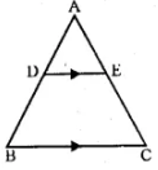Solution:-
(i) We have given that: DE || BC
We have to prove that, ∆ADE and ∆ABC are similar
∠A = ∠A … [common angle for both triangles]
∠ADE = ∠ABC … [because corresponding angles are equal]
Therefore, ∆ADE ~ ∆ABC … [AA axiom]
(ii) From (i) we proved that, ∆ADE ~ ∆ABC
Then, AD/AB = AB/AC = DE/BC
(½ BD)/ ((½BD) + BD) = DE/4.5
(½ BD)/ ((3/2)BD) = DE/4.5
½ × (2/3) = DE/4.5
1/3 = DE/4.5
Therefore, DE = 4.5/3
DE = 1.5 cm
(iii) From the question it is given that, area of ∆ABC = 18 cm^2
Then, area of ∆ADE/area of ∆ABC = DE2/BC2
area of ∆ADE/18 = (1/3)2  = 1/9
area of ∆ADE = 18 × 1/9
So, area of trapezium DBCE = area of ∆ABC – area of ∆ADE
= 18 – 2
= 16 cm2
hence, the area of trapezium DBCE is 16cm

8. In the given figure, AB and DE are perpendicular to BC.
(i) Prove that ∆ABC ~ ∆DEC
(ii) If AB = 6 cm: DE = 4 cm and AC = 15 cm, calculate CD.
(iii) Find the ratio of the area of ∆ABC : area of ∆DEC.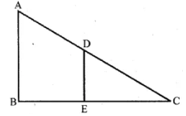Solution:-
We have given that:
(i) Consider the ∆ABC and ∆DEC,
∠ABC = ∠DEC … [both angles are equal to 90o]
∠C = ∠C … [common angle for both triangles]
Therefore, ∆ABC ~ ∆DEC … [by AA axiom]
(ii) AC/CD = AB/DE
Corresponding sides of similar triangles are proportional
15/CD = 6/4
CD = (15 × 4)/6
CD = 60/6
CD = 10 cm
Hence, the length of CD=10 cm
(iii) we know that, area of ∆ABC/area of ∆DEC = AB2/DE2
area of ∆ABC/area of ∆DEC = 62/42
area of ∆ABC/area of ∆DEC = 36/16
area of ∆ABC/area of ∆DEC = 9/4
Therefore, the ratio of the area of ∆ABC : area of ∆DEC is 9∶ 4.

9. In the adjoining figure, ABC is a triangle. DE is parallel to BC and AD/DB = 3/2,
(ii) Prove that ∆DEF is similar to ∆CBF. Hence, find EF/FB.
(iii) What is the ratio of the areas of ∆DEF and ∆CBF?Solution:-
We have given that:
(i) To find the ratios AD/AB,DE/BC,
From the question it is given that, AD/DB = 3/2
Now add 1 for both LHS and RHS we get,
(DB/AD) + 1 = (2/3) + 1
From the figure (DB + AD) = AB
Now, consider the ∆ADE and ∆ABC,
∠ADE = ∠B … [corresponding angles are equal]
∠AED = ∠C … [corresponding angles are equal]
Therefore, ∆ADE ~ ∆ABC … [by AA similarity]
Then, AD/AB = DE/BC = 3/5
(ii) Now consider the ∆DEF and ∆CBF
∠EDF = ∠BCF … [because alternate angles are equal]
∠DEF = ∠FBC … [because alternate angles are equal]
∠DFE = ∠ABFC … [because vertically opposite angles are equal]
Therefore, ∆DEF ~ ∆CBF
So, EF/FB = DE/BC = 3/5
(iii) we have to find the ratio of the areas of ∆DEF and ∆CBF,
We know that, Area of ∆DFE/Area of ∆BFC = DE2/BC2
Area of ∆DFE/Area of ∆BFC = DE2/BC2
Area of ∆DFE/Area of ∆BFC = (3/5)2
Area of ∆DFE/Area of ∆BFC = 9/25
Therefore, the ratio of the areas of ∆DEF and ∆CBF is 9: 25.

10. In ∆ABC,AP∶ PB = 2∶ 3.PO is parallel to BC and is extended to Q so that CQ is parallel to BA. Find:
(i) area ∆APO : area ∆ABC.
(ii) area ∆APO : area ∆CQO.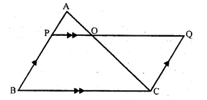Solution:-
We have given that:
PB = 2: 3
PO is parallel to BC and is extended to Q so that CQ is parallel to BA.
(i) we have to find the area ∆APO: area ∆ABC,
Then,
∠A = ∠A … [common angles for both triangles]
∠APO = ∠ABC … [because corresponding angles are equal]
Then,  ∆APO ~ ∆ABC … [AA axiom]
We know that, area of ∆APO/area of ∆ABC = AP2/AB2
= AP2/(AP + PB)2
= 22/(2 + 3)2
= 4/52
= 4/25
Therefore, area ∆APO: area ∆ABC is 4: 25
(ii) we have to find the area ∆APO: area ∆CQO
Then, ∠AOP = ∠COQ … [because vertically opposite angles are equal]
∠APQ = ∠OQC … [because alternate angles are equal]
Therefore, area of ∆APO/area of ∆CQO = AP2/CQ2
area of ∆APO/area of ∆CQO = AP2/PB2
area of ∆APO/area of ∆CQO = 22/32
area of ∆APO/area of ∆CQO = 4/9
Therefore, area ∆APO: area ∆CQO is 4: 9

11.(a) In the figure (i) given below, ABCD is a trapezium in which AB || DC and AB = 2 CD. Determine the ratio of the areas of ∆AOB and ∆COD.Solution:-
We have given that:
ABCD is a trapezium in which AB || DC and AB = 2 CD,
Then, ∠OAB = ∠OCD … [because alternate angles are equal]
∠OBA = ∠ODC
Then, ∆AOB ~ ∆COD
So, area of ∆AOB/area of ∆COD = AB2/CD2
= (2CD)2/CD2 … [because AB = 2 CD]
= 4CD2/CD2
= 4/1
Therefore, the ratio of the areas of ∆AOB and ∆COD is 4: 1.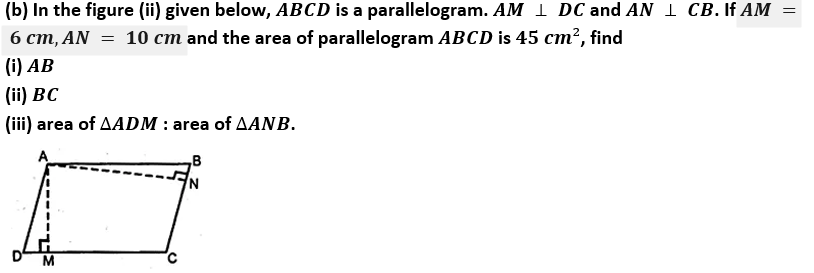Solution:-
We have given that:
ABCD is a parallelogram, AM ⊥ DC and AN ⊥ CB
AM = 6 cm
AN = 10 cm
The area of parallelogram ABCD is 45 cm²
Then, area of parallelogram ABCD = DC × AM = BC × AN
45 = DC × 6 = BC × 10
(i) DC = 45/6
Divide both numerator and denominator by 3 we get,
= 15/2
EB/AB = 3/5
Therefore, EF∶ AD is 3: 5
(ii) we have to find area of ∆BEF: area of ∆ABD,
Then, area of ∆BEF/area of ∆ABD = (EF)2 /(AD)2
area of ∆BEF/area of ∆ABD = 32/52
= 9/25
Therefore, area of ∆BEF: area of ∆ABD is 9: 25
(iii) From (ii) area of ∆ABD/area of ∆BEF = 25/9
25 area of ∆BEF = 9 area of ∆ABD
25(area of ∆ABD – area of trapezium AEFD) = 9 area of ∆ABD
25 area of ∆ABD – 25 area of trapezium AEFD = 9 area of ∆ABD
25 area of trapezium AEFD = 25 area of ∆ABD – 9 area of ∆ABD
25 area of trapezium AEFD = 16 area of ∆ABD
area of ∆ABD/area of trapezium AEFD = 25/16
Therefore, area of ∆ABD : area of trapezium AFED = 25: 16
(iv) Now we have to find area of ∆FEO : area of ∆OBC
So, consider ∆FEO and ∆OBC,
∠EOF = ∠BOC … [because vertically opposite angles are equal]
∠F = ∠OBC … [because alternate angles are equal]
∆FEO ~ ∆OBC
Then, area of FEO/area of ∆OBC = EF2/BC2
Therefore, area of ∆FEO: area of ∆OBC = 9: 25.

12. In the adjoining figure, ABCD is a parallelogram. P is a point on BC such that BP∶ PC = 1∶ 2 and DP produced meets AB produced at Q. If area of ∆CPQ = 20 cm², find(i) area of ∆BPQ.
(ii) area ∆CDP.
(iii) area of parallelogram ABCD.
Solution:-
We have given that: ABCD is a parallelogram.
BP: PC = 1: 2
area of ∆CPQ = 20 cm²
Construction: draw QN perpendicular CB and Join BN.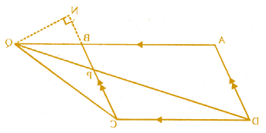Then, area of ∆BPQ/area of ∆CPQ = ((½BP) × QN)/((½PC) × QN)
= BP/PC = ½
(i) So, area ∆BPQ = ½ area of ∆CPQ
= ½ × 20
Therefore, area of ∆BPQ = 10 cm2
(ii) Now we have to find area of ∆CDP,
Consider the ∆CDP and ∆BQP,
Then, ∠CPD = ∠QPD … [because vertically opposite angles are equal]
∠PDC = ∠PQB … [because alternate angles are equal]
Therefore, ∆CDP ~ ∆BQP … [AA axiom]
area of ∆CDP/area of ∆BQP = PC2/BP2
area of ∆CDP/area of ∆BQP = 22/12
area of ∆CDP/area of ∆BQP = 4/1
area of ∆CDP = 4 × area ∆BQP
Therefore, area of ∆CDP = 4 × 10
= 40 cm2
(iii) We have to find the area of parallelogram ABCD,
Area of parallelogram ABCD = 2 area of ∆DCQ
= 2 area (∆DCP + ∆CPQ)
= 2 (40 + 20)cm2
= 2 × 60 cm2
= 120 cm2
Therefore, the area of parallelogram ABCD is 120 cm2 .

13. (a) In the figure (i) given below, DE || BC and the ratio of the areas of ∆ADE and trapezium DBCE is 4∶ 5. Find the ratio of DE∶ BC.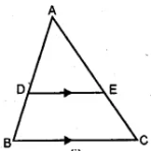Solution:-
We have given that:
DE || BC
The ratio of the areas of ∆ADE and trapezium DBCE is 4∶ 5
Now, consider the ∆ABC and ∆ADE
∠A = ∠A … [common angle for both triangles]
∠D = ∠B and
∠E = ∠C  … [because corresponding angles are equal]
So, area of ∆ADE/area of ∆ABC = (DE)2 /(BC)2 … [equation (i)]
Then, area of ∆ADE/area of trapezium DBCE = 4/5
area of trapezium DBCE/area of ∆ADE = 5/4
Add 1 for both LHS and RHS we get,
(area of trapezium DBCE/area of ∆ADE) + 1 = (5/4) + 1
(area of trapezium DBCE + area of ∆ADE)/area of ∆ADE = (5 + 4)/4
area of ∆ABC/area of ∆ADE = 9/4
area of ∆ADE/area of ∆ABC = 4/9
From equation (i),
area of ∆ADE/area of ∆ABC = (DE)2 /(BC)2
area of ∆ADE/area of ∆ABC = (DE)2 /(BC)2  = 42/92
area of ∆ADE/area of ∆ABC = (DE)2 /(BC)2= 2/3
Therefore, DE: BC = 2: 3

(b) In the figure (ii) given below, AB || DC and AB = 2 DC. If AD = 3 cm,BC = 4 cm and AD,BC produced meet at E, find (i) ED (ii) BE (iii) area of ∆EDC : area of trapezium ABCD.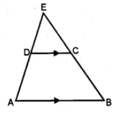Solution:-
We have given that:
AB || DC
AB = 2 DC,AD = 3 cm,BC = 4 cm
Now consider ∆EAB,
EA/DA = EB/CB = AB/DC = 2DC/DC = 2/1
(i) EA = 2,DA = 2 × 3 = 6 cm
Then, ED = EA – DA
= 6 – 3
= 3 cm
(ii) EB/CB = 2/1
EB = 2 CB
EB = 2 × 4
EB = 8 cm
(iii) Now, consider the ∆EAB,DC || AB
So, ∆EDC ~ ∆EAB
Therefore, area of ∆EDC/area of ∆ABE = DC2/AB2
area of ∆EDC/area of ∆ABE = DC2 /(2DC)2
area of ∆EDC/area of ∆ABE = DC2/4DC2
area of ∆EDC/area of ∆ABE = ¼
Therefore, area of ABE = 4 area of ∆EDC
Then, area of ∆EDC + area of trapezium ABCD = 4 area of ∆EDC
Area of trapezium ABCD = 3 area of ∆EDC
So, area of ∆EDC/area of trapezium ABCD = 1/3
Therefore, area of ∆EDC: area of trapezium ABCD = 1: 3

14. (a) In the figure given below, ABCD is a trapezium in which DC is parallel to AB. If AB = 9 cm,DC = 6 cm and BB = 12 cm., find (i) BP (ii) the ratio of areas of ∆APB and ∆DPC.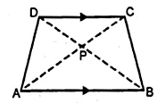Solution:-
We have given that:
DC is parallel to AB
AB = 9 cm,DC = 6 cm and BB = 12 cm
(i) Consider the ∆APB and ∆CPD
∠APB = ∠CPD … [because vertically opposite angles are equal]
∠PAB = ∠PCD … [because alternate angles are equal]
So, ∆APB ~ ∆CPD
Then, BP/PD = AB/CD
BP/(12 – BP) = 9/6
6BP = 108 – 9BP
6BP + 9BP = 108
15BP = 108
BP = 108/15
Therefore, BP = 7.2 cm
(ii) We know that, area of ∆APB/area of ∆CPD = AB2/CD2
area of ∆APB/area of ∆CPD = 92/62
area of ∆APB/area of ∆CPD = 81/36
By dividing both numerator and denominator by 9, we get,
area of ∆APB/area of ∆CPD = 9/4
Therefore, the ratio of areas of ∆APB and ∆DPC is 9: 4

(b) In the figure given below, ∠ABC = ∠DAC and AB = 8 cm,AC = 4 cm,AD = 5 cm. (i) Prove that ∆ACD is similar to ∆BCA (ii) Find BC and CD (iii) Find the area of ∆ACD : area of ∆ABC.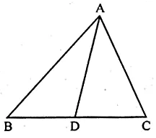Solution:-
We have given that:
∠ABC = ∠DAC
AB = 8 cm,AC = 4 cm,AD = 5 cm
(i) Now, consider ∆ACD and ∆BCA
∠C = ∠C … [common angle for both triangles]
∠ABC = ∠CAD … [from the question]
So, ∆ACD ~ ∆BCA … [by AA axiom]
(ii) AC/BC = CD/CA = AD/AB
4/BC = 5/8
BC = (4 × 8)/5
BC = 32/5
BC = 6.4 cm
CD/4 = 5/8
CD = (4 × 5)/8
CD = 20/8
CD = 2.5 cm
Hence, the length of CD=2.5 cm
(iii) from (i) we proved that, ∆ACD ~ ∆BCA
area of ∆ACB/area of ∆BCA = AC2/AB2
= 4^2/8^2
= 16/64
By dividing both numerator and denominator by 16, we get,
= ¼
Therefore, the area of ∆ACD : area of ∆ABC is 1: 4.

15. ABC is a right angled triangle with ∠ABC = 90°. D is any point on AB and DE is perpendicular to AC. Prove that:
(ii) If AC = 13 cm,BC = 5 cm and AE = 4 cm. Find DE and AD.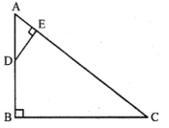Solution:-
We have given that:
∠ABC = 90°
AB and DE is perpendicular to AC
(i) Consider the ∆ADE and ∆ACB,
∠A = ∠A … [common angle for both triangle]
∠B = ∠E … [both angles are equal to 90o]
(ii) from (i) we proved that, ∆ADE ~ ∆ACB
So, AE/AB = AD/AC = DE/BC … [equation (i)]
Consider the ∆ABC, is a right angle triangle
From Pythagoras theorem, we have
AC2  = AB2 + BC2
132  = AB2 + 52
169 = AB2 + 25
AB2 = 169 – 25
AB2  = 144
AB = √144
AB = 12 cm
Consider the equation (i),
Now, take AE/AB = DE/BC
4/12 = DE/5
1/3 = DE/5
DE = (5 × 1)/3
DE = 5/3
DE = 1.67 cm
(iii) Now, we have to find area of ∆ADE∶ area of quadrilateral BCED,
We know that, Area of ∆ADE = ½ × AE × DE
= ½ × 4 × (5/3)
= 10/3 cm2
Then, area of quadrilateral BCED = area of ∆ABC – area of ∆ADE
= ½ × BC × AB – 10/3
= ½ × 5 × 12 – 10/3
= 1 × 5 × 6 – 10/3
= 30 – 10/3
= (90 – 10)/3
= 80/3 cm2
So, the ratio of area of ∆ADE : area of quadrilateral BCED = (10/3)/(80/3)
= (10/3) × (3/80)
= (10 × 3)/(3 × 80)
= (1 × 1)/(1 × 8)
= 1/8
Therefore, area of ∆ADE : area of quadrilateral BCED is 1: 8.

16. Two isosceles triangles have equal vertical angles and their areas are in the ratio 7: 16. Find the ratio of their corresponding height.
Solution:-
We have given that:
Consider the two isosceles triangle PQR and XYZ,∠P = ∠X … [from the question]
So, ∠Q + ∠R = ∠Y + ∠Z
∠Q = ∠R and ∠Y = ∠Z [because opposite angles of equal sides]
Therefore, ∠Q = ∠Y and ∠R = ∠Z
∆PQR ~ ∆XYZ
Then, area of ∆PQR/area of ∆XYZ = PM2/XN2 … [from corollary of theorem]
PM^2/XN2= 7/16
PM/XN = √7/√16
PM/XN = √7/4
Therefore, ratio of PM: DM = √7: 4

17. On a map drawn to a scale of 1∶ 250000, a triangular plot of land has the following measurements : AB = 3 cm,BC = 4 cm and ∠ABC = 90°. Calculate
(i) the actual length of AB in km.
(ii) the area of the plot in sq.km:
Solution:-
We have given that:
Map drawn to a scale of 1: 250000
AB = 3 cm,BC = 4 cm and ∠ABC = 90°
(i) We have to find the actual length of AB in km.
Let us assume scale factor K = 1: 250000
K = 1/250000
Then, length of AB of actual plot = 1/k × length of AB on the map
= (1/(1/250000)) × 3
= 250000 × 3
To covert cm into km divide by 100000
= (250000 × 3)/(100 × 1000)
= 15/2
length of AB of actual plot = 7.5 km
(ii) We have to find the area of the plot in sq. km
Area of plot on the map = ½ × AB × BC
= ½ × 3 × 4
= ½ × 12
= 1 × 6
= 6 cm^2
Then, area of actual plot = 1/k2 × 6 cm2
= 2500002  × 6
To covert cm into km divide by (100000)2
= (250000 × 250000 × 6)/(100000 × 100000)
= (25/4) × 6
= 75/2
= 37.5 km2

18. On a map drawn to a scale of 1∶ 25000, a rectangular plot of land, ABCD has the following measurements AB = 12 cm and BG = 16 cm. Calculate:
(i) the distance of a diagonal of the plot in km.
(ii) the area of the plot in sq.km.
Solution:-
We have given that:
Map drawn to a scale of 1: 25000
AB = 12 cm,BG = 16 cm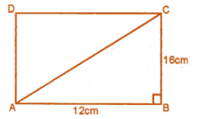Consider the ∆ABC,
From the Pythagoras theorem,
AC2  = AB2  + BC2
AC = √(AB2  + BC2)
= √((12)2  + (16)2 )
= √144  + 256
= √400
= 20 cm
Then, area of rectangular plot ABCD = AB × BC
= 12 × 16
= 192 cm2
(i) We have to find the distance of a diagonal of the plot in km. .
Let us assume scale factor K = 1: 25000
K = 1/25000
Then, length of AB of actual plot = 1/k × length of diagonal of rectangular plot
= (1/(1/25000)) × 3
= 25000 × 20
To covert cm into km divide by 100000
= (25000 × 20)/(100 × 1000)
= 5 km
(ii) We have to find the area of the plot in sq. km.
Then, area of actual plot = 1/k2  × 192 cm2
= 250002  × 192
To covert cm into km divide by (100000)2
= (25000 × 25000 × 192)/(100000 × 100000)
= 12 km2

19. The model of a building is constructed with the scale factor 1∶ 30.
(i) If the height of the model is 80 cm, find the actual height of the building in metres.
(ii) If the actual volume of a tank at the top of the building is 27 m³, find the volume of the tank on the top of the model.
Solution:-
We have given that:
The model of a building is constructed with the scale factor 1∶ 30
So, Height of the model/Height of actual building = 1/30
(i) Given, the height of the model is 80 cm
Then, 80/H = 1/30
H = (80 × 30)
H = 2400 cm
H = 2400/100
H = 24 m
(ii) Given, the actual volume of a tank at the top of the building is 27 m³
Volume of model/Volume of tank = (1/30)3
V/27 = 1/27000
V = 27/27000
V = 1/1000 m3
Therefore, Volume of model = 1000 cm3

20. A model of a ship is made to a scale of 1∶ 200.
(i) If the length of the model is 4 m, find the length of the ship.
(ii) If the area of the deck of the ship is 160000 m², find the area of the deck of the model.
(iii) If the volume of the model is 200 liters, find the volume of the ship in m³. (100 liters = 1 m³)
Solution:-
We have given that: a model of a ship is made to a scale of 1∶ 200
(i) Given, the length of the model is 4 m
Then, length of the ship = (4 × 200)/1
= 800 m
(ii) Given, the area of the deck of the ship is 160000 m²
Then, area of deck of the model = 160000 × (1/200)2
= 160000 × (1/40000)
= 4 m²
(iii) Given, the volume of the model is 200 liters
Then, Volume of ship = 200 × (200/1)3
= 200 × 8000000
= (200 × 8000000)/100
= 1600000 m3

Chapter Test

1. In the adjoining figure, ∠1 = ∠2 and ∠3 = ∠4. Show that PT x QR = PR × ST.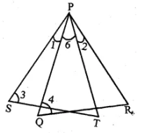Solution:-
We have given that:
∠1 = ∠2 and ∠3 = ∠4
We have to prove that, PT x QR = PR x ST
Given, ∠1 = ∠2
Adding ∠6 to both LHS and RHS we get,
∠1 + ∠6 = ∠2 + ∠6
∠SPT = ∠QPR
Consider the ∆PQR and ∆PST,
From above ∠SPT = ∠QPR
∠3 = ∠4
Therefore, ∆PQR ~ ∆PST
So, PT/PR = ST/QR
By cross multiplication we get,
PT× QR = PR × ST
Hence, proved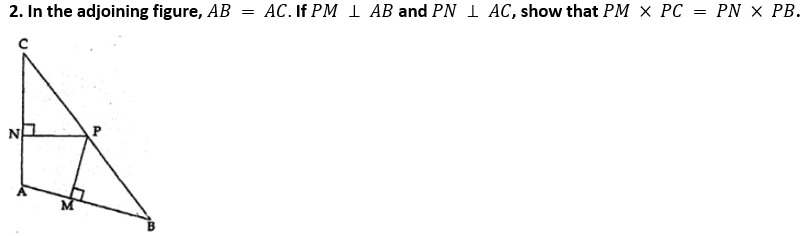Solution:-
We have given that:
AB = AC. If PM ⊥ AB and PN ⊥ AC
We have to show that, PM x PC = PN x PB
Consider the ∆ABC,
AB = AC … [given]
∠B = ∠C
Then, consider ∆CPN and ∆BPM
∠N = ∠M … [both angles are equal to 90°
∠C = ∠B … [from above]
Therefore, ∆CPN ~ ∆BPM … [from AA axiom]
So, PC/PB = PN/PM
By cross multiplication we get,
PC × PM = PN × PB
Hence proved

3.(a) In the figure given below. ∠AED = ∠ABC. Find the values of x and y.Solution:-
We have given that:
∠AED = ∠ABC
∠AED = ∠ABC … [from the figure]
∠A = ∠A … [common angle for both triangles]
Therefore, ∆ABC ~ ∆ADE … [by AA axiom]
3/(4 + 2) = y/10
3/6 = y/10
By cross multiplication we get,
y = (3 × 10)/6
y = 30/6
y = 5
Now, consider AB/AE = BC/DE
(3 + x)/4 = 10/y
Substitute the value of y,
(3 + x)/4 = 10/5
By cross multiplication,
5(3 + x) = 10 x 4
15 + 5x = 40
5x = 40 – 15
5x = 25
X = 25/5
x = 5
Therefore, the value of x = 5 cm and y = 5 cm

(b) In the figure given below, CD = ½ AC,B is mid-point of AC and E is mid-point of DF. If BF || AG, prove that :
(i) CE || AG
(ii) 3 ED = GD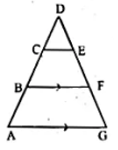Solution:-
We have given that:
CD = ½ AC
BF || AG
(i) We have to prove that, CE || AG
Consider, CD = ½ AC
AC = 2BC … [because from the figure B is mid-point of AC]
So, CD = ½ (2BC)
CD = BC
Hence, CE || BF … [equation (i)]
Given, BF || AG … [equation (ii)]
By comparing the results of equation (i) and equation (ii) we get,
CE || AG
(ii) We have to prove that, 3 ED = GD
Consider the ∆AGD,
CE || AG … [above it is proved]
AD = AB + BC + DC
= DC + DC + DC
= 3DC
So, ED/GD = DC/(3DC)
ED/GD = 1/(3(1))
ED/GD = 1/3
3ED = GD
Hence proved, 3ED = GD

4. In the adjoining figure, 2 AD = BD, E is mid-point of BD and F is mid-point of AC and EC || BH. Prove that:
(i) DF || BH
(ii) AH = 3 AF.Solution:-
According to question,
We have given that,
2 AD = BD,EC || BH
(i) Given, E is mid-point of BD
2DE = BD … [equation (i)]
2AD = BD … [equation (ii)]
From equation (i) and equation (ii) we get,
Also given that, F is mid-point of AC
DF || EC … [equation (iii)]
Given, EC || BH … [equation (iv)]
By comparing equation (iii) and equation (iv) we get,
DF || BH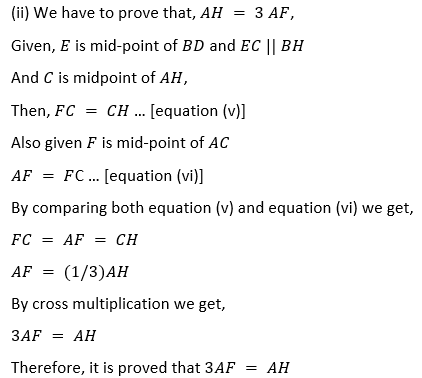5. In a ∆ABC,D and E are points on the sides AB and AC respectively such that DE || BC. If AD = 2.4 cm, AE = 3.2 cm,DE = 2 cm and BC = 5 cm, find BD and CE.
Solution:-
We have given that: In a ∆ABC,D and E are points on the sides AB and AC respectively.
DE || BC
AD = 2.4 cm,AE = 3.2 cm,DE = 2 cm and BC = 5 cm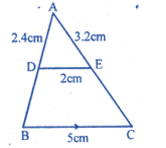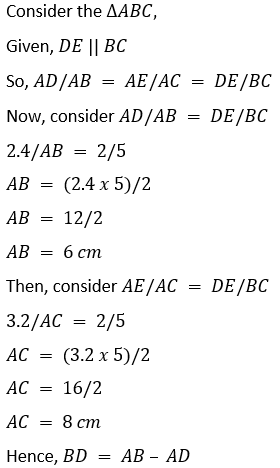= 6 – 2.4
= 3.6 cm
CE = AC – AE
= 8 – 3.2
= 4.8 cm
Hence, the length of CE=4.8 cm

6. In a ∆ABC,D and E are points on the sides AB and AC respectively such that AD = 5.7cm,BD = 9.5cm,AE = 3.3cm and AC = 8.8cm. Is DE || BC? Justify your answer.
Solution:-
We have given that:
In a ∆ABC,D and E are points on the sides AB and AC respectively.
AD = 5.7cm,BD = 9.5cm,AE = 3.3cm and AC = 8.8cm
Consider the ∆ABC,
EC = AC – AE
= 8.8 – 3.3
= 5.5 cm
= 57/95
By dividing both numerator and denominator by 19 we get,
= 3/5
AE/EC = 3.3/5.5
= 33/55
By dividing both numerator and denominator by 11 we get,
= 3/5
Therefore, DE || BC

7. If the areas of two similar triangles are 360 cm² and 250 cm² and if one side of the first triangle is 8 cm, find the length of the corresponding side of the second triangle.
Solution:-
We have given that: the areas of two similar triangles are 360 cm² and 250 cm².
one side of the first triangle is 8 cm
So, PQR and XYZ are two similar triangles,So, let us assume area of ∆PQR = 360 cm2,QR = 8 cm
And area of ∆XYZ = 250 cm2
Assume YZ = a
We know that, area of ∆PQR/area of ∆XYZ = QR2/yz2
360/250 = (8)2/a2
360/250 = 64/a2
By cross multiplication we get,
a2  = (250 ×64)/3608. In the adjoining figure, D is a point on BC such that ∠ABD = ∠CAD. If AB = 5 cm,AC = 3 cm and AD = 4 cm, find
(i) BC
(ii) DC
(iii) area of ∆ACD∶ area of ∆BCA.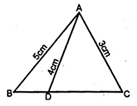Solution:-
We have given that:
AB = 5 cm,AC = 3 cm and AD = 4 cm
Now, consider the ∆ABC and ∆ACD
∠C = ∠C … [common angle for both triangles]
∠ABC = ∠CAD … [from the question]
So, ∆ABC ~ ∆ACD
Then, AB/AD = BC/AC = AC/DC
5/4 = BC/3
BC = (5 × 3)/4
BC = 15/4
BC = 3.75 cm
Hence, the length of BC=3.5 cm

5/4 = 3/DC
DC = (3 × 4)/5
DC = 12/5
DC= 2.4 cm
Hence, the length of DC=2.4 cm
(iii) Consider the ∆ABC and ∆ACD
∠CAD = ∠ABC … [from the question]
∠ACD = ∠ACB … [common angle for both triangle]
Therefore, ∆ACD ~ ∆ABC
Then, area of ∆ACD/area of ∆ABC = AD2/AB2
= 42/52
= 16/25
Therefore, area of ∆ACD : area of ∆BCA is 16: 25.

9. In the adjoining figure, the diagonals of a parallelogram intersect at O.OE is drawn parallel to CB to meet AB at E, find area of ∆AOE : area of parallelogram ABCD.Solution:-
We have given that:
From the given figure,
The diagonals of a parallelogram intersect at O.
OE is drawn parallel to CB to meet AB at E.
In the figure four triangles have equal area.
So, area of ∆OAB = ¼ area of parallelogram ABCD
Then, O is midpoint of AC of ∆ABC and DE || CB
E is also midpoint of AB
Therefore, OE is the median of ∆AOB
Area of ∆AOE = ½ area of ∆AOB
= ½ × ¼ area of parallelogram ABCD
= 1/8 area of parallelogram ABCD
So, area of ∆AOE/area of parallelogram ABCD = 1/8
Therefore, area of ∆AOE: area of parallelogram ABCD is 1: 8.

10. In the given figure, ABCD is a trapezium in which AB || DC.If 2AB = 3DC, find the ratio of the areas of ∆AOB and ∆COD.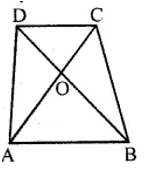Solution:-
We have given that,
ABCD is a trapezium in which AB || DC.If 2AB = 3DC.
So, 2AB = 3DC
AB/DC = 3/2
Now, consider ∆AOB and ∆COD
∠AOB = ∠COD … [because vertically opposite angles are equal]
∠OAB = ∠OCD … [because alternate angles are equal]
Therefore, ∆AOB ~ ∆COD … [from AA axiom]
Then, area of ∆AOB/area of ∆COD = AB2/DC2
= 32/22
= 9/4
Therefore, the ratio of the areas of ∆AOB and ∆COD is 9: 4

11. In the adjoining figure, ABCD is a parallelogram. E is mid-point of BC.DE meets the diagonal AC at O and meet AB (produced) at F. Prove that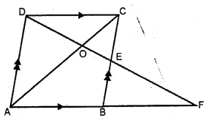Solution:-
We have given that:
ABCD is a parallelogram. E is mid-point of BC.
DE meets the diagonal AC at O.
(i) Now consider the ∆AOD and ∆EDC,
∠AOD = ∠EOC … [because Vertically opposite angles are equal]
∠OAD = ∠OCB … [because alternate angles are equal]
Therefore, ∆AOD ~ ∆EOC
Then, OA/OC = DO/OE = AD/EC = 2EC/EC
OA/OC = DO/OE = 2/1
Therefore, OA: OC = 2: 1
(ii) From (i) we proved that, ∆AOD ~ ∆EOC
So, area of ∆OEC/area of ∆AOD = OE2/DO2
area of ∆OEC/area of ∆AOD = 12/22
area of ∆OEC/area of ∆AOD = ¼
Therefore, area of ∆OEC: area of ∆AOD is 1: 4.

12. A model of a ship is made to a scale of 1: 250 calculate:
(i) The length of the ship, if the length of model is 1.6 m.
(ii) The area of the deck of the ship, if the area of the deck of model is 2.4 m2.
(iii) The volume of the model, if the volume of the ship is 1 km3.
Solution:-
We have given that: a model of a ship is made to a scale of 1∶ 250
(i) Given, the length of the model is 1.6 m
Then, length of the ship = (1.6 × 250)/1
= 400 m
(ii) Given, the area of the deck of the ship is 2.4 m²
Then, area of deck of the model = 2.4 × (1/250)2
= 1,50,000 m2  = 4 m
(iii) Given, the volume of the model is 1 km3
Then, Volume of ship = (1/2503) × 1 km3
= 1/(250)3  × 1000
= 43
= 64 m3
Therefore, volume of ship is 64 m3.